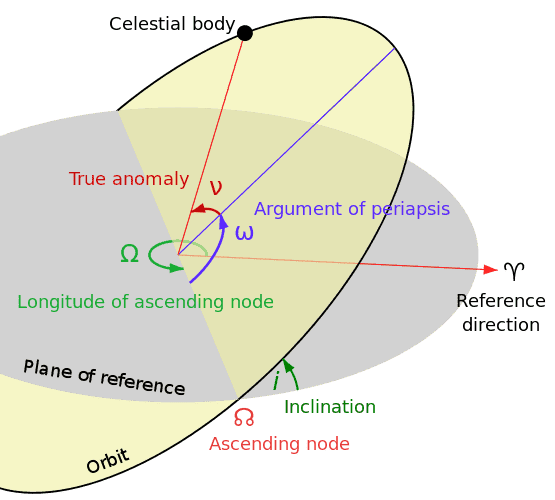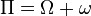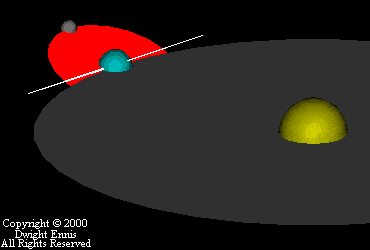# Relativistic orbital precession in practice

• A
I'm interested how was measured the famous Mercury's precession: 43'' / cy.

How it has been measured?
where is the angle 43'' - in this image:http://en.wikibooks.org/wiki/Astrodynamics/Classical_Orbit_Elements

The preccesion is about the periapse, so it's defined wrt the node:therefore if the node changes, the peri moves too.
So, what is the precession of nodes for the Mercury?

The orbit is a precessing ellipse. The orbit ellipse remains in the same plane as always. It has to, Gravity in this case is still a central force so there is no motion out of the plane. At one time I thought the plane of orbit precessed in space. This is certainly not true. the orbit plane remains fixed in space. It is the ellipse that rotates in that plane. It sweeps out a figure that in general does not close, even after many many orbits.

If I understand correctly the relativistic precession is about the absidal precession of the flat orbit only, yes?

But the Mercury's orbit is not flat, because it's inclined strongly,
about 7 degrees, thus I'm still confused.

How and where has been the angle 43 arcsec/cy observed, measured?

1. in an absolute sense, what means the 43 is the angle between two eccentrity vectors: measured in a hundred years of time span?
2. wrt the node only? But the node moves too, therefore I don't know what the angle represents at all.
3. ?

More precisely.

http://en.wikipedia.org/wiki/Tests_of_general_relativity

531.63 ±0.69 Gravitational tugs of the other planets
0.0254 Oblateness of the Sun (quadrupole moment)
42.98 ±0.04 General relativity
574.64±0.69 Total
574.10±0.65

My question is: wrt of what these values have been measured?

More:
if I would to realise a numerical simulation of the solar system;
so, what, where I should to measure, to discovery these angles?

PeterDonis
Mentor
If I understand correctly the relativistic precession is about the absidal precession of the flat orbit only, yes?

But the Mercury's orbit is not flat, because it's inclined strongly,

"Inclined" is relative; the "inclination" just means the plane of Mercury's orbit is inclined with respect to the plane of the Earth's orbit. But all of the precession angles for a given orbit are measured in the plane of that orbit.

wrt of what these values have been measured?

The direct measurement is the total precession. The values for gravitational perturbations due to the other planets and for the Sun's oblateness are calculated based on independent measurements. The 43 arcsec/century is what is left over when you subtract the calculated values for gravitational perturbations and solar oblateness from the measured value. The fact that that 43 left over matches the GR prediction to a good accuracy is why we view this as an experimental confirmation of GR.

Last edited:
"Inclined" is relative; the "inclination" just means the plane of Mercury's orbit is inclined with respect to the plane of the Earth's orbit. But all of the precession angles for a given orbit are measured in the plane of that orbit.

That means the precession is measured wrt the node, which moves and backward... like a spinning top, or the moon orbit:So, the measured angle for the apse motion is rather artificial,
because without the nodal precession it would be different.

I even can suppose the 43''/cy anomaly is a simply a geometric effect of the nodal precession:

the angle of apse anomaly = the nodal precession * sin i

The inclination is about 6 degrees, thus: sin i = 0.1;
the nodal precession is about 430 arcsec/cy, I think.

Therefore the additional apse shift, observed due to the node motion,
is equal to: 43 arcsec/cy, which has been identified as the GR precession!

PeterDonis
Mentor
That means the precession is measured wrt the node

No, it doesn't. The diagram and animated graphic you showed are talking about the plane of some object's orbit relative to some chosen reference plane. That is not what is being referred to when we talk about the precession of Mercury's perihelion. We are talking about a phenomenon that happens entirely within Mercury's orbital plane; it has nothing to do with the orientation of Mercury's orbital plane relative to anything else.

http://physics.ucr.edu/~wudka/Physics7/Notes_www/node98.html

Note Figure 7.20 on the above page; it shows the precession as it happens in the plane of the orbit.

https://en.wikipedia.org/wiki/Tests_of_general_relativity#Perihelion_precession_of_Mercury

Ibix
So, if I'm understanding Peter right, on the diagram in the OP the precession is ##d\omega/dt## and the 43" is the difference between the Newtonian prediction of that value and the measured value

No, it doesn't. The diagram and animated graphic you showed are talking about the plane of some object's orbit relative to some chosen reference plane. That is not what is being referred to when we talk about the precession of Mercury's perihelion. We are talking about a phenomenon that happens entirely within Mercury's orbital plane; it has nothing to do with the orientation of Mercury's orbital plane relative to anything else.

You probably omitted one simple fact:
the nodal precession is very real, like any rotation is:
the orbital plane rotates really, thus wrt any reference frame!

But I'm strongly dissapointed, because I still can't understand
how the 43''/cy was discovered/observed in the real astronomy:

what has been measured, and next subtracted to get the 43'' of a discrepancy?

PeterDonis
Mentor
on the diagram in the OP the precession is ##d\omega/dt##

Yes.

the 43" is the difference between the Newtonian prediction of that value and the measured value

Yes.

"what has been measured, and next subtracted to get the 43'' of a discrepancy?"

I have in mind:
what has been measured, and next subtracted to get the 574'' of the peri motion?

Where is this angle: between of which lines, points, vectors?

And a second, very simple question:
what is the nodal precession of the Mercury's orbit?

Last edited:
PeterDonis
Mentor
the orbital plane rotates really, thus wrt any reference frame!

Not quite. You can always choose a frame in which the orbital plane is fixed. But it is true that relative changes in the orbital planes of different bodies, with respect to each other, will be present in any frame, if they exist at all. See further comments below.

Where is this angle: between of which lines, points, vectors?

See post #10.

what is the nodal precession of the Mercury's orbit?

I have not found any numbers for the nodal precession of any of the planets online; only for satellites. I suspect this is because the nodal precessions of the planets, in the standard solar system reference frame, are too small to have been measured if they exist at all, whereas the nodal precessions of satellites with respect to their primaries are large enough to be easily observed.

Not quite. You can always choose a frame in which the orbital plane is fixed. But it is true that relative changes in the orbital planes of different bodies, with respect to each other, will be present in any frame, if they exist at all.

The nodal precession is not less real than the apsidal one!

Look at this:
http://en.wikipedia.org/wiki/Precession

The commonly known precession of a spinning disk is just called as: a nodal precession, in the astronomy!
The whole orbital plane rotates along some axis, like a spinning flat/planar disk, when a torque is only applied.

I have not found any numbers for the nodal precession of any of the planets online; only for satellites. I suspect this is because the nodal precessions of the planets, in the standard solar system reference frame, are too small to have been measured if they exist at all, whereas the nodal precessions of satellites with respect to their primaries are large enough to be easily observed.

Maybe that's the cause of the whole confusion about the anomalistic precession.
So, I just predict the nodal precession of the Mercury is equal to: 430''/cy +/- 10%.

PeterDonis
Mentor
The nodal precession is not less real than the apsidal one!

As I said, the "nodal precession" in the sense of a rate of change of a particular coordinate quantity depends on the coordinates you choose. Given any body in orbit about some other body, you can always choose coordinates such that the plane of the orbit is always the x-y plane. But in those coordinates, the plane which is called the "reference plane" in the diagram you gave in your OP will have a inclination that changes with time. So the relative orientation of the reference plane and the plane of the orbit does change, independent of your choice of coordinates. You are just used to working in coordinates in which the reference plane is fixed, so the relative change in orientation of a body's orbital plane vs. the reference plane in those coordinates is the same as the coordinate rate of change.

the Mercury's node moves with a speed:
-0.12214182 degs/cy = 439.7'' / cy.

Hm, interesting, so it is certainly large enough to be measured. And you will also notice that the longitude of perihelion rate of change is 0.15940013 degs/cy, or 573.8 arcsec/cy. So that is where the measured value for Mercury's perihelion precession comes from; and subtracting the calculated values for the Newtonian perturbations of the other planets and for solar oblateness gives the 43 arcsec/cy left over that is accounted for by the GR prediction.

As I said, the "nodal precession" in the sense of a rate of change of a particular coordinate quantity depends on the coordinates you choose. Given any body in orbit about some other body, you can always choose coordinates such that the plane of the orbit is always the x-y plane. But in those coordinates, the plane which is called the "reference plane" in the diagram you gave in your OP will have a inclination that changes with time. So the relative orientation of the reference plane and the plane of the orbit does change, independent of your choice of coordinates. You are just used to working in coordinates in which the reference plane is fixed, so the relative change in orientation of a body's orbital plane vs. the reference plane in those coordinates is the same as the coordinate rate of change.

You have in the astronomy the reference plane which is relative - incorrect, because it's just the ecliptic - the Earth orbit, which precesses too!
In the physics, mechanics the reference plane must be and is fixed in space, thus it's the invariant plane of the Solar System, at least!

Any orbit can precess in an absolute - newtonian sense, simply in a space.
And you will also notice that the longitude of perihelion rate of change is 0.15940013 degs/cy, or 573.8 arcsec/cy. So that is where the measured value for Mercury's perihelion precession comes from; and subtracting the calculated values for the Newtonian perturbations of the other planets and for solar oblateness gives the 43 arcsec/cy left over that is accounted for by the GR prediction.

This is nothing progress in my investigations,
because there is still undefined the angle: 574'' - wrt of what it was measured?
Maybe to the moving Mercury's node, within the ecliptic, which is inconstant also?!

So, I can conclude only: nothing has been measured in fact!

PeterDonis
Mentor
You have in the astronomy the reference plane which is relative - incorrect, because it's just the ecliptic - the Earth orbit

In other words, the ecliptic is the "reference plane" if you choose coordinates in which the ecliptic is the reference plane. This is perfectly consistent with what I said.

which precesses too!

Precesses relative to what?

In the physics, mechanics the reference plane must be and is fixed in space

Fixed in space relative to what?

The mistake you are making is to think that the term "fixed in space" has some absolute meaning. It doesn't. There is no absolute space.

there is still undefined the angle: 574'' - wrt of what it was measured?

Once again, have you read post #10? Or have you read the document you yourself linked to, which explains what the "longitude of perihelion" measurement given in that same document--the one that gives a measurement of 574 arcsec/century, as shown in the same table in the same document from which you took the nodal precession measurement--is?

I can conclude only: nothing has been measured in fact!

No, the correct conclusion is that you have not read the document that you yourself linked to. See above.

phyzguy
What is measured is the position of Mercury in the sky as seen from Earth. This can be measured very accurately, to a small fraction of an arcsecond. As Mercury approaches perihelion it speeds up, then slows down again as it passes perihelion. So you need to know where the perihelion is to accurately predict its location on the sky. From a model of the Earth's orbit and a model of Mercury's orbit, you can predict exactly where in the sky it should be at any given time. You then vary the orbital elements of the orbits until you find those that best match the observations. By doing this for multiple planets, you can determine the Earth's orbital parameters very precisely, and from the observations of where Mercury is in the sky, you can accurately determine the elements of Mercury's orbit, including the location of the perihelion. From how much the perihelion position changes from orbit to orbit, you can determine the rate of precession.

•Dale
In other words, the ecliptic is the "reference plane" if you choose coordinates in which the ecliptic is the reference plane. This is perfectly consistent with what I said.

The real ecliptic isnt used as a reference plane, because it still changes.
Only a temporaliry ecliptic can be used there, thus these numbered epochs in the astronomy: J1900, J1950, and now J2000.
http://en.wikipedia.org/wiki/Epoch_(reference_date)

The mistake you are making is to think that the term "fixed in space" has some absolute meaning. It doesn't. There is no absolute space.

The absolute space is a fundamental element in any theory, especially in the Newton's dynamics, which is necessary to discover the newtonian precession: 532''/cy.

Or have you read the document you yourself linked to, which explains what the "longitude of perihelion" measurement given in that same document--the one that gives a measurement of 574 arcsec/century, as shown in the same table in the same document from which you took the nodal precession measurement--is?

This is rather impossible Le Verrier did know the number: 439.7'' / cy - the Mercury's nodal precession, therefore his measurements are completely useless.

What is measured is the position of Mercury in the sky as seen from Earth. This can be measured very accurately, to a small fraction of an arcsecond. As Mercury approaches perihelion it speeds up, then slows down again as it passes perihelion. So you need to know where the perihelion is to accurately predict its location on the sky. From a model of the Earth's orbit and a model of Mercury's orbit, you can predict exactly where in the sky it should be at any given time. You then vary the orbital elements of the orbits until you find those that best match the observations. By doing this for multiple planets, you can determine the Earth's orbital parameters very precisely, and from the observations of where Mercury is in the sky, you can accurately determine the elements of Mercury's orbit, including the location of the perihelion. From how much the perihelion position changes from orbit to orbit, you can determine the rate of precession.

I want to measure directly the angle of precession.. say: in a numerical simulation of the solar system: 574, or 532.
So, where I can discovery, measure this fantastic angle - precisely?

PeterDonis
Mentor
The real ecliptic isnt used as a reference plane

It isn't in the coordinates used in the caltech source you linked to--there different coordinates are used for different epochs. But one could also adopt coordinates in which the real ecliptic is always fixed. In those coordinates the other measurements given in the source would be numerically different because they would be referred to a different coordinate system.

The absolute space is a fundamental element in any theory

It isn't in GR, so this statement is obviously false.

especially in the Newton's dynamics, which is necessary to discover the newtonian precession: 532''/cy.

No, all that is necessary for that is to use the Newtonian limit of GR. You don't need to assume absolute space for that.

This is rather impossible Le Verrier did know the number: 439.7'' / cy - the Mercury's nodal precession, therefore his measurements are completely useless.

I have no idea what you are trying to say here. If you are somehow under the impression that the measurements given in the caltech source you linked to are from Le Verrier, that is, um, not the case.

where I can discovery, measure this fantastic angle - precisely?

The same way as the measurements were made that are given in the caltech source you linked to. You appear to believe that the nodal precession measurements given there are real measurements. The perihelion precession measurement of 574 arcsec/century for Mercury comes from the same source.

PeterDonis
Mentor
At this point the OP question has been answered and the discussion is just going around in circles. Thread closed.

Mark44
Mentor
and the discussion is just going around in circles
Or in ellipses...